﻿ 基于FPGA的宽光谱光纤法珀干涉信号的稀疏采样系统的研究

# 基于FPGA的宽光谱光纤法珀干涉信号的稀疏采样系统的研究Research on High Speed Sparse Sampling System for Wide Spectral Fiber Fabry Sensor Signals Based on FPGA

Abstract: The existing fast demodulation model of the wide-spectrum fiber fabry interference signal can demodulate the signal based on the reduction of the spectral sampling points of two orders of magnitude to ensure the original accuracy, which lays the foundation for greatly improving the demodulation speed of wide-spectrum fiber fabry system. In this paper, a high-speed sparse sampling hardware system with 40 sampling points for wide-spectrum interference signals is designed. By comparing the results of dense sampling and sparse sampling of the same Fabry-spectrum interference signal, the conclusion that the sparse sampling hardware system can reproduce the wide-spectrum interference signal well is obtained. This is of great significance for the application of wide-spectrum fiber fabry sensors in high-speed monitoring.

1. 引言

2. 稀疏采样的可行性分析

2.1. 光纤法珀传感器系统原理Figure 1. Fiber fabry sensor measurement system and sensor structure diagram

${I}_{r}\left(\lambda \right)=2R\left[1-\mathrm{cos}\left(\frac{\text{4π}}{\lambda }L\right)\right]\cdot I\left(\lambda \right)$ (1)

2.2. 稀疏采样的可行性分析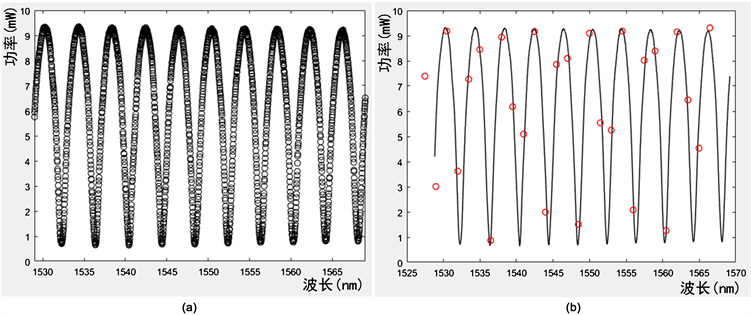Figure 2. Two ways to sample wide-spectrum interference signals. (a) Dense sampling; (b) sparse sampling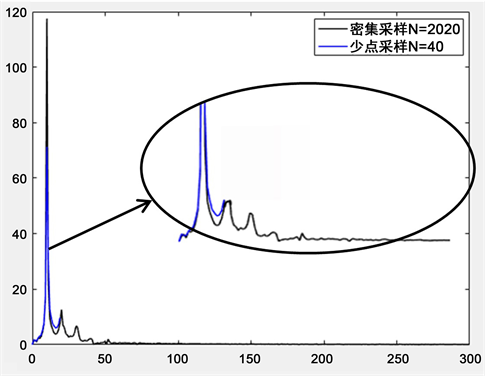Figure 3. The results of FT transformation for spectral data of dense sampling and sparse sampling.

3. 宽光谱干涉信号稀疏采集系统总体设计方案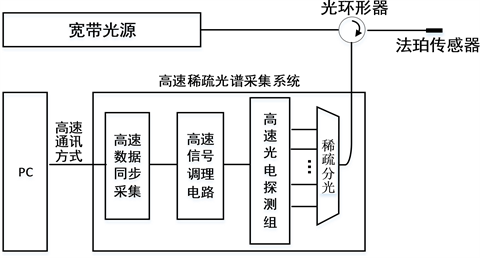Figure 4. Total design of high-speed sparse acquisition hardware system for wide-spectrum interference signals

3.1. 光谱稀疏分光

3.2. 光谱稀疏采样点数的理论依据

${I}_{r}\left(\lambda \right)=2R\mathrm{cos}\left(2\text{π}{\Delta }_{OPD}k\right)\cdot {I}_{0}\left(\lambda \right)$ (2)

$\frac{{F}_{s}}{{\Delta }_{OPD}}=2~10倍$ (3)

$\frac{1}{10{\Delta }_{OPD}}\le \Delta \delta \le \frac{1}{2{\Delta }_{OPD}}$ (4)Table 1. Number of sampling points of fiber fabry sensor under different cavity lengths

3.3. 模拟电路调理

$A={R}_{2}\cdot \frac{{R}_{4}}{{R}_{3}}$ (5)

${B}_{1}=\sqrt{\frac{GB{P}_{1}}{2\text{π}{R}_{2}{C}_{j}}}$ (6)

${B}_{2}=\frac{GB{P}_{2}}{{A}_{2}}$ (7)

$B=\frac{1}{\sqrt{\frac{1}{{B}_{1}^{2}}+\frac{1}{{B}_{2}^{2}}}}$ (8)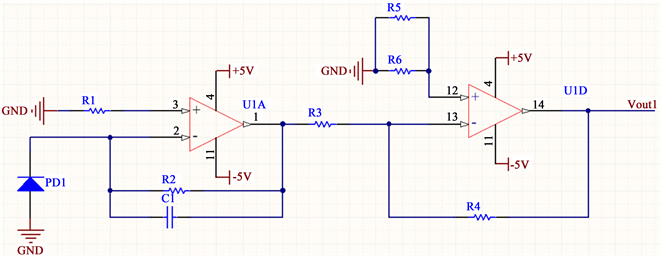Figure 5. Analog circuit conditioning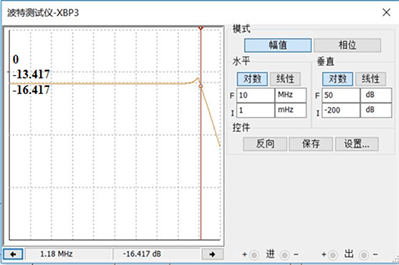Figure 6. The simulation results of analog circuit’s total gain and total bandwidth by Multisim

3.4. 高速同步采集

3.5. 高速通讯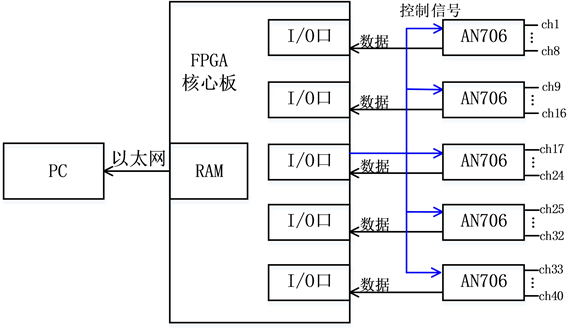Figure 7. FPGA realizes synchronous acquisition and communication with PC by Ethernet

3.6. 搭建宽光谱干涉信号高速稀疏采集硬件系统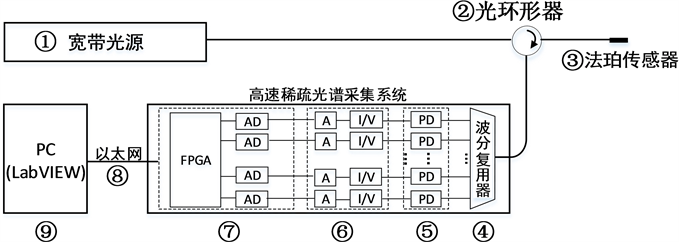Figure 8. High-speed sparse acquisition hardware system for wide-spectrum interference signals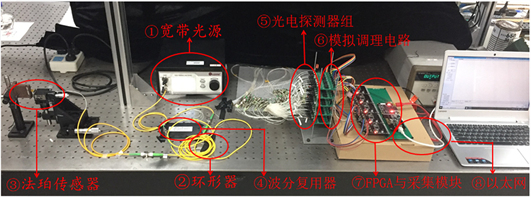Figure 9. The physical picture of high-speed sparse acquisition hardware system

4. 实验

4.1. 实验系统搭建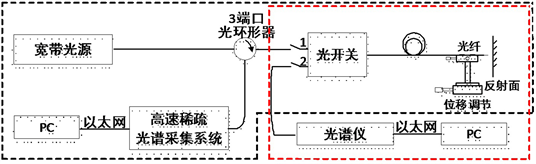Figure 10. Experimental system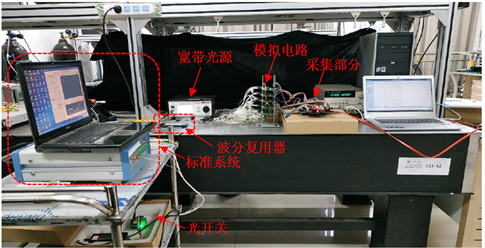Figure 11. The physical picture of experimental system

4.2. 采样功能验证实验过程及实验结果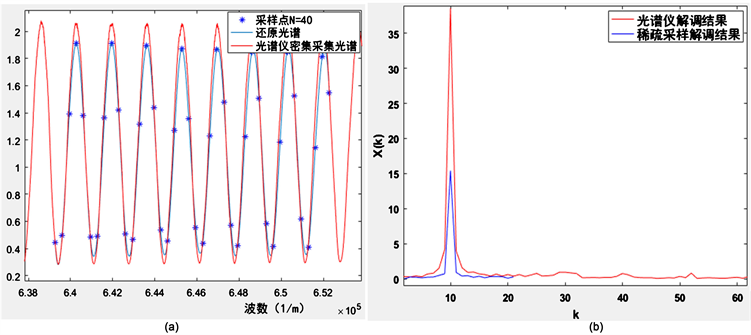Figure 12. The experiment results of sparse sampling. (a) Sparse sampling and dense sampling recurring spectral signals; (b) sparse sampling and dense sampling FT transformation results

5. 结论

NOTES

*通讯作者。

 陈伟民, 雷小华, 张伟, 等. 光纤法布里–珀罗传感器研究进展[J]. 光学学报, 2018, 38(3): 132-145.

 章鹏, 朱永, 唐晓初, 陈伟民. 基于傅里叶变换的光纤法布里–珀罗传感器解调研究[J]. 光学学报, 2005, 25(2): 186-189.

 Yu, Z.H. and Wang, A.B. (2016) Fast Demodulation Algorithm for Multiplexed Low-Finesse Fabry-Perot Interferometers. Journal of Lightwave Tech-nology, 34, 1015-1019.
https://doi.org/10.1109/JLT.2016.2515943

 怀鑫. 光纤传感网络中微弱光信号的检测[D]. 北京: 北京交通大学, 2017.

 杨小优, 唐政维, 周平等. PIN光电探测器低噪声前置放大电路设计[J]. 电子质量, 2012(3): 43-45.

 胡涛, 司汉英. 光电探测器前置放大电路设计与研究[J]. 光电技术应用, 2010, 25(1): 52-55.

 宋涛, 张斌, 罗倩倩. 光电转换电路的设计与优化[J]. 光电技术应用, 2010, 25(6): 46-48.

 张国雄, 李醒飞. 测控电路[M]. 北京: 机械工业出版社, 2011.

 张贵清, 朱磊, 颜露新, 等. 基于FPGA的多路同步实时数据采集方案设计与实现[J]. 测控技术, 2005, 24(12): 26-29.

 林长青, 孙胜利. 基于FPGA的多路高速数据采集系统[J]. 电测与仪表, 2005, 42(5): 52-54.

 胡波, 李鹏. 异步FIFO在FPGA与DSP通信中的运用[J]. 电子科技, 2011, 24(3): 53.

Top